Precalculus : Evaluate Expressions With Rational Exponents

Example Questions

Example Question #11 : Rational Exponents

Simplify the expression: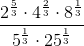.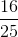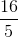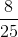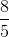Explanation:

First, you can begin to simplfy the numerator by converting all 3 expressions into base 2.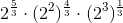, which simplifies to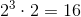For the denominator, the same method applies. Convert the 25 into base 5, and when simplified becomes simply 5.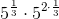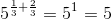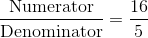Example Question #2 : Evaluate Expressions With Rational Exponents

Evaluate the following expression using knowledge of the properties of exponents: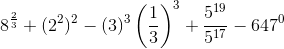Explanation:

Let's work through this equation involving exponents one term at a time. The first term we see is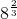, for which we can apply the following property: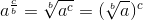So if we plug our values into the formula for the property, we get: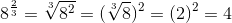Because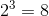. Our next term is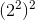, for which we'll need the property: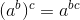Using the values for our term, we have: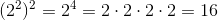The third term of the equation is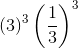, for which the quickest way to evaluate would be using the following property: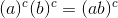Using the values from our term, this gives us: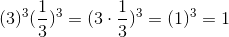The next property we will need to consider for our fourth term is given below: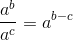If we plug in the corresponding values from our term, we get: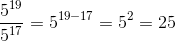Finally, our last term requires knowledge of the following simple property: Any number raised to the power of zero is 1. With this in mind, our last term becomes: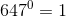Rewriting the equation with all of the values we've just evaluated, we obtain our final answer: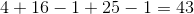Example Question #1 : Evaluate Expressions With Rational Exponents

Evaluate the following expression and solve for.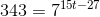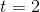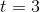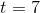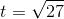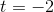Explanation:

To solve this problem, recall that you can set exponents equal to eachother if they have the same base.

See below: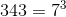So, we have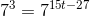Because both sides of this equation have a base of seven, we can set the exponents equal to eachother and solve for t.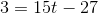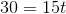Example Question #1 : Evaluate Expressions With Rational Exponents

Solve for.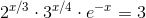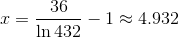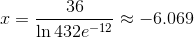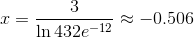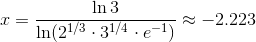Explanation:

We begin by taking the natural log of the equation: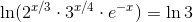Simplifying the left side of the equation using the rules of logarithms gives: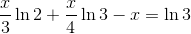We group the x terms to get: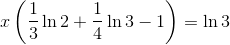We reincorporate the exponents into the logarithms and use the identity property of the natural log to obtain: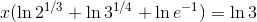We combine the logarithms using the multiplication/sum rule to get: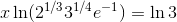We then solve for x: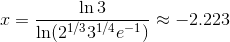Example Question #2 : Evaluate Expressions With Rational Exponents

Solve for.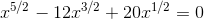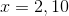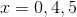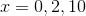Explanation:

We begin by factoring out the term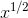to get: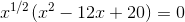This equation gives our first solution: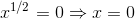Then we check for more solutions: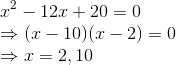Therefore our solution isExample Question #1 : Evaluate Expressions With Rational Exponents

Evaluate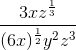when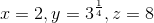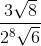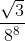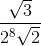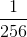Explanation:

Remember the denominator of a rational exponent is equivalent to the index of a root.

This should simplify quite nicely.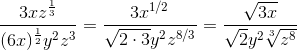Whenit gives us,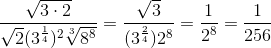Example Question #61 : Exponential And Logarithmic Functions

What is the value of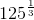?15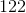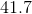Explanation:

What does an exponent of one-third mean? Consider our expression and raise it to the third power.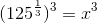Simplifying, we get: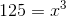Thus, we are looking for a number that when cubed, we get. Thus, we are discussing the cube root of, orAll Precalculus Resources